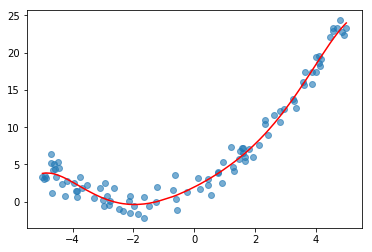# 机器学习实战之训练模型-多项式回归（一）

$${y=β_{0}+β_{1}x+\varepsilon }$$

$${y=β _{0}+β_{1}x+β_{2}x^{2}+\varepsilon }$$

$${y=β _{0}+β_{1}x+β_{2}x^{2}+β_{3}x^{3}+\cdots +β_{n}x^{n}+\varepsilon }$$

## 一、单个特征值

### 1.1 生成演示数据

import numpy as np
import matplotlib.pyplot as plt

X = 10 * np.random.rand(100,1) - 5
X = np.sort(X,axis=0) #为了方便作图，X和y的每一个组合的顺序没有关系的
y = 0.5* X ** 2 + 2 * X + 2 + np.random.randn(100,1)
plt.scatter(X,y,c='green', alpha=0.6)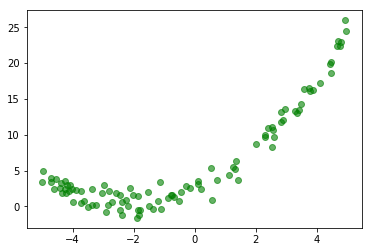### 1.2 生成新的特征

from sklearn.preprocessing import PolynomialFeatures
pf = PolynomialFeatures(degree = 2,include_bias = False)
#include_bias：默认为True。如果为True的话，那么就会有常量为1的那一项。
#interaction_only： 默认为False，如果指定为True，那么就不会有特征自己和自己结合的项。
X_new = pf.fit_transform(X)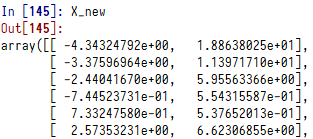### 1.3 用新的特征进行回归

from sklearn.linear_model import LinearRegression
reg = LinearRegression()
reg.fit(X_new,y)
reg.intercept_
reg.coef_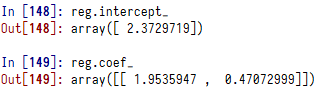### 1.4 拟合效果

plt.scatter(X,y,c='green', alpha=0.6)
plt.plot(X, reg.predict(X_new), color='r')
plt.show()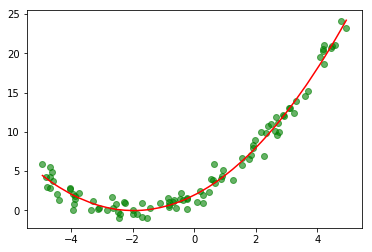## 二、多个特征值

### 2.1 生成演示数据

X1 = 10 * np.random.rand(100,1) - 5
X2 = 2 * np.random.rand(100,1) - 9
y = 2 * X1 + 3 * X2 + 4 * X1**2 + 5 * X1*X2 + 6 * X2**2 + 7 + np.random.randn(100,1)
X = pd.DataFrame(np.c_[X1,X2])
X.columns=['x1','x2']

### 2.2 生成新的特征

pf = PolynomialFeatures(degree=2).fit(X)
X_new = pf.transform(X)
clumns_list = pf.get_feature_names(X.columns)
features = pd.DataFrame(X_new, columns=clumns_list)

X_new是这样的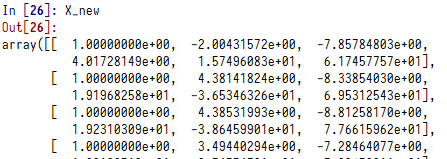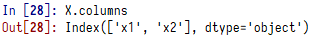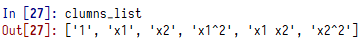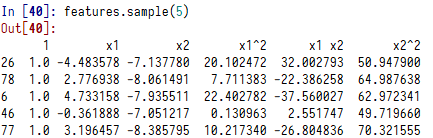### 2.3 用新的特征进行回归

reg = LinearRegression()
reg.fit(features,y)
reg.intercept_
reg.coef_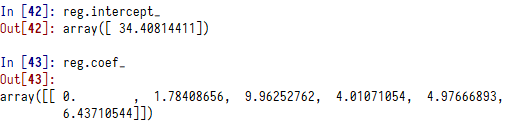!!多个特征我们无法在平面画出拟合图。

### 2.4 数据标准化

from sklearn.preprocessing import StandardScaler

scaler = StandardScaler().fit(X_new)
X_new_std = scaler.transform(X_new)
features_std = pd.DataFrame(X_new_std, columns=clumns_list)
reg = LinearRegression()
reg.fit(features_std,y)
reg.intercept_

reg.coef_

## 三、Pipeline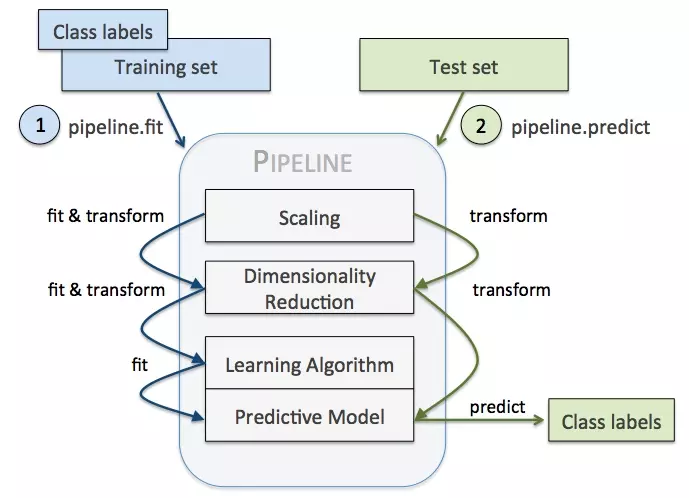pipeline最后一步如果有predict()方法我们才可以对pipeline使用fit_predict()，同理，最后一步如果有transform()方法我们才可以对pipeline使用fit_transform()方法。

### 3.1 生成模拟数据

X = 10 * np.random.rand(100,1) - 5
X = np.sort(X,axis=0) ##为了方便作图，X和y的每一个组合的顺序没有关系的
y = 0.5* X ** 2 + 2 * X + 2 + np.random.randn(100,1)

### 3.2 建立模型

poly_reg = Pipeline([
("poly", PolynomialFeatures(degree=6)),
("std_scaler", StandardScaler()),
("lin_reg", LinearRegression())
])

### 3.3 拟合曲线

poly_reg.fit(X, y)
y_predict = poly_reg.predict(X)
plt.scatter(X,y, alpha=0.6)
plt.plot(X, y_predict, color='r')
plt.show()